# Load: q=100lb/in BP1: Determine normal and transverse shear stress on a simply supported rectangular 5-ft beam...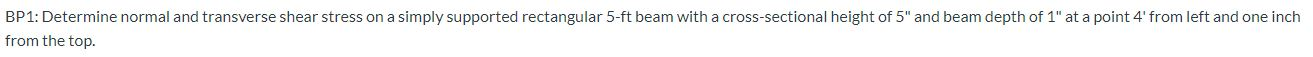Load: q=100lb/in

BP1: Determine normal and transverse shear stress on a simply supported rectangular 5-ft beam with a cross-sectional height of 5" and beam depth of 1" at a point 4' from left and one inch from the top.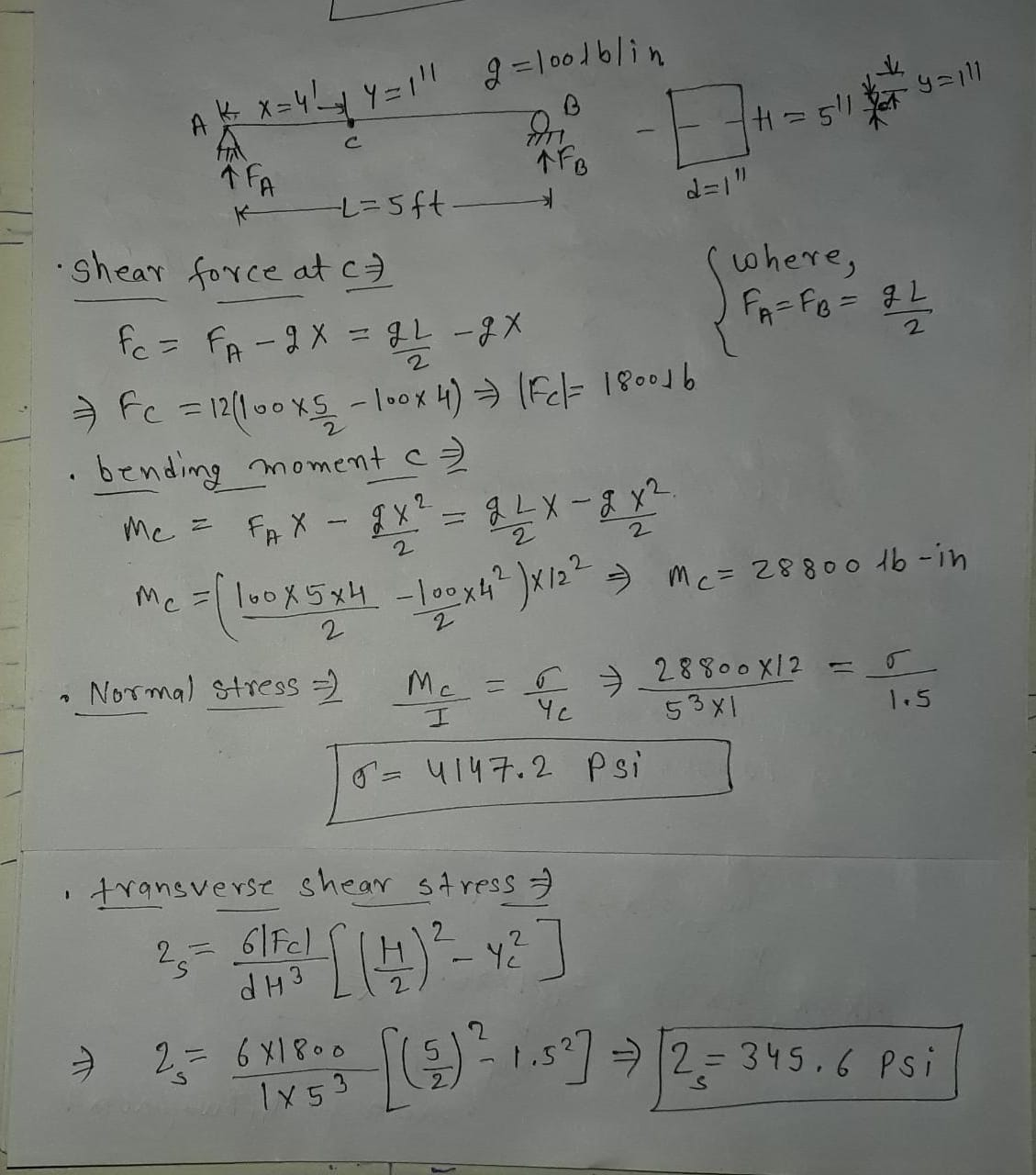##### Add Answer to: Load: q=100lb/in BP1: Determine normal and transverse shear stress on a simply supported rectangular 5-ft beam...
Similar Homework Help Questions
• ### 14.54 A simply supported rectangular beam with a span of 6 ft has a concentrated load of 4.1 kip ...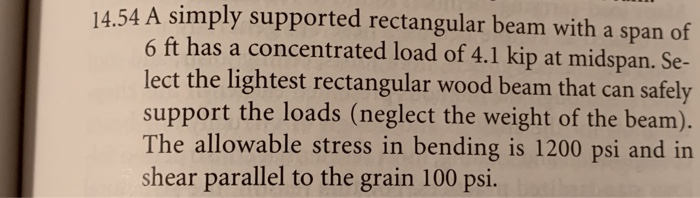14.54 A simply supported rectangular beam with a span of 6 ft has a concentrated load of 4.1 kip at midspan. Se- lect the lightest rectangular wood beam that can safely support the loads (neglect the weight of the beam). The allowable stress in bending is 1200 psi and in shear parallel to the grain 100 psi 14.54 A simply supported rectangular beam with a span of 6 ft has a concentrated load of 4.1 kip at midspan. Se- lect...

• ### 5. (20 Points) For the loading on the simply supported beam shown below. a) What is...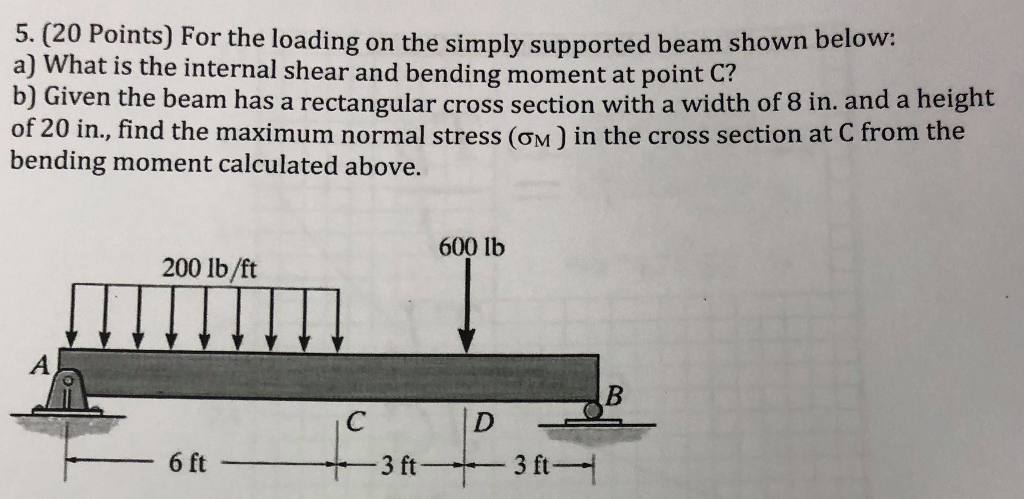5. (20 Points) For the loading on the simply supported beam shown below. a) What is the internal shear and bending moment at point C? b) Given the beam has a rectangular cross section with a width of 8 in. and a height of 20 in., find the maximum normal stress (om) in the cross section at C from the bending moment calculated above. 600 lb 200 lb/ft

• ### A simply supported beam is subjected to a load applied as shown in the figure. Draw the shear for...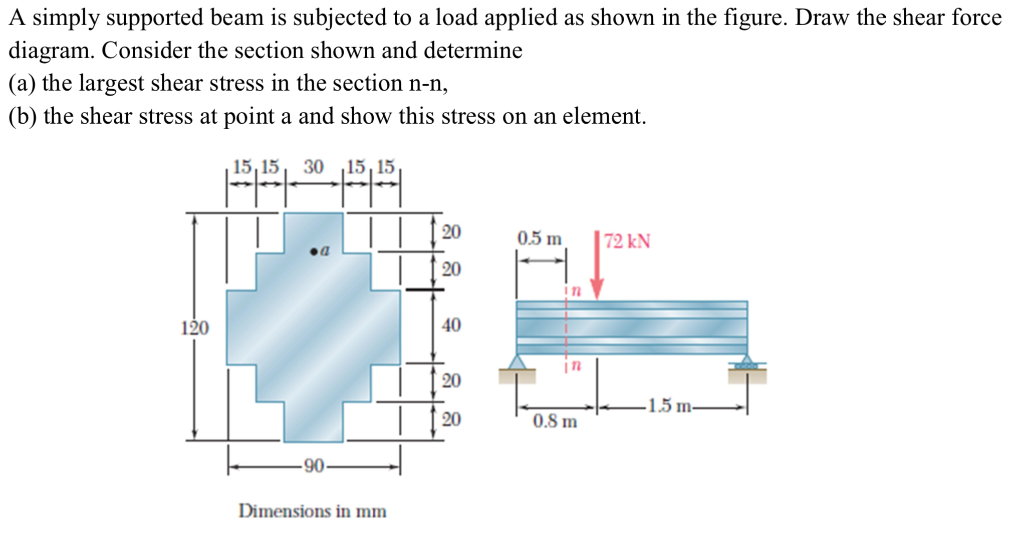A simply supported beam is subjected to a load applied as shown in the figure. Draw the shear force diagram. Consider the section shown and determine (a) the largest shear stress in the section n-n, (b) the shear stress at point a and show this stress on an element. 15,15 30 15,15 20 I n 40 120 15m 0.8 m Dimensions in mm A simply supported beam is subjected to a load applied as shown in the figure. Draw the...

• ### 2. A 30 ft long simply supported beam supports a uniformly distributed load of 2 kips/ft...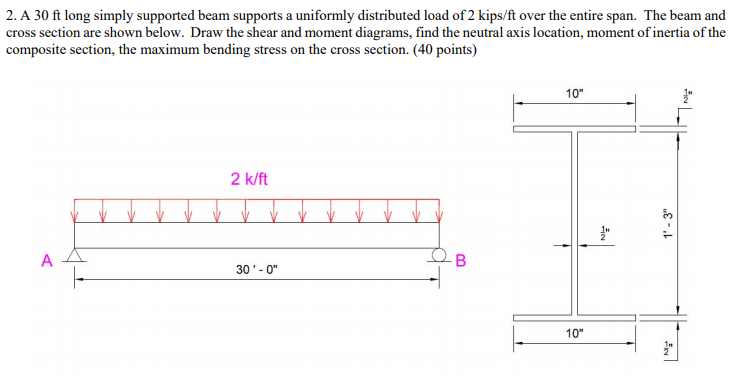2. A 30 ft long simply supported beam supports a uniformly distributed load of 2 kips/ft over the entire span. The beam and cross section are shown below. Draw the shear and moment diagrams, find the neutral axis location, moment of inertia of the composite section, the maximum bending stress on the cross section. (40 points) 10" 2 k/ft 1-3" 30'-0"

• ### A5.2 m long simply supported wood beam carries a uniformly distributed load of 12.9 kN/m, as...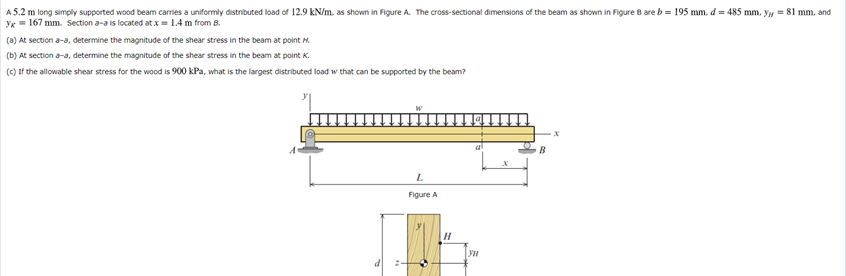A5.2 m long simply supported wood beam carries a uniformly distributed load of 12.9 kN/m, as shown in Figure A. The cross-sectional dimensions of the beam as shown in Figure Bare b = 195 mm, d = 485 mm. yy = 81 mm, and yx = 167 mm. Section 3-a is located at x = 1.4 m from B. (a) At section a-a, determine the magnitude of the shear stress in the beam at point H. (b) At section a-3,...

• ### Simply supported rectangular beam shown is 18in. wide and has an effective depth of 40in. Beam supports a factored load of 7.63 kips/ft on a clear span of 26.5ft. Given load includes the beam's s...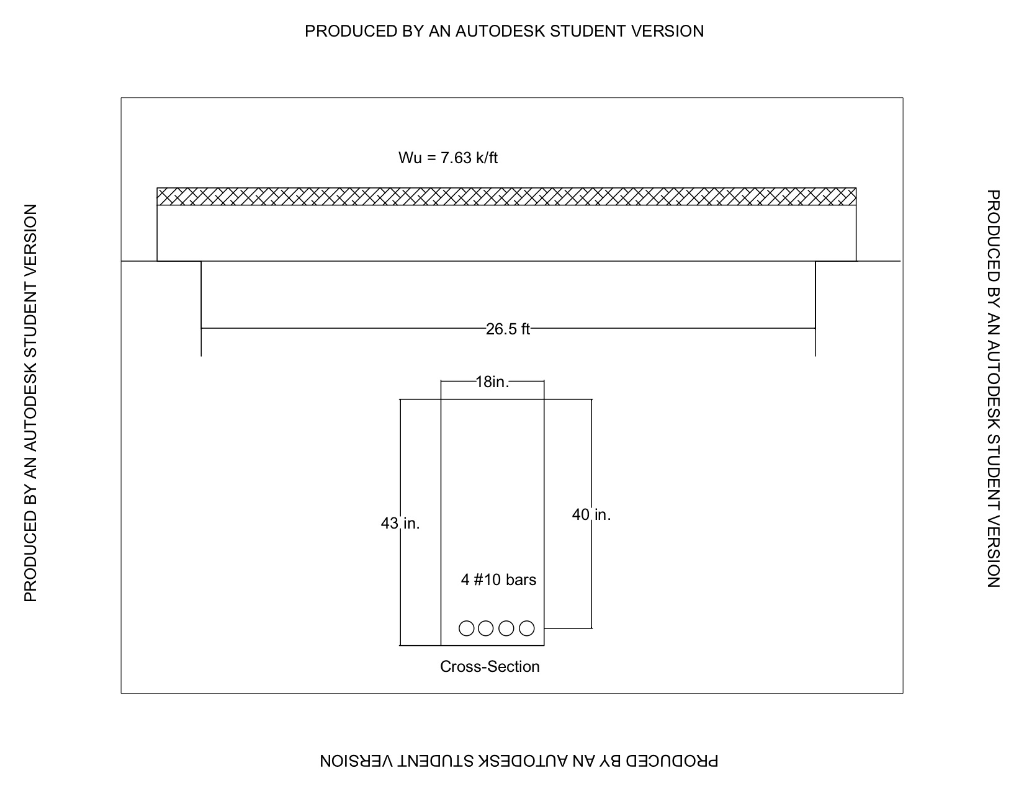Simply supported rectangular beam shown is 18in. wide and has an effective depth of 40in. Beam supports a factored load of 7.63 kips/ft on a clear span of 26.5ft. Given load includes the beam's self weight. Design the web reinforcement. Use f'c = 4,000psi and fy = 60,000psi. Show work and equations used. Draw shear diagram and stirrup design. PRODUCED BY AN AUTODESK STUDENT VERSION Wu 7.63 k/ft 26.5 ft 18in 40 in 43 in. 4 #10 bars Cross-Section PRODUCED...

• ### Chapter 15, Supplemental Question 121 The simply supported beam supports a uniformly distributed load of w-300...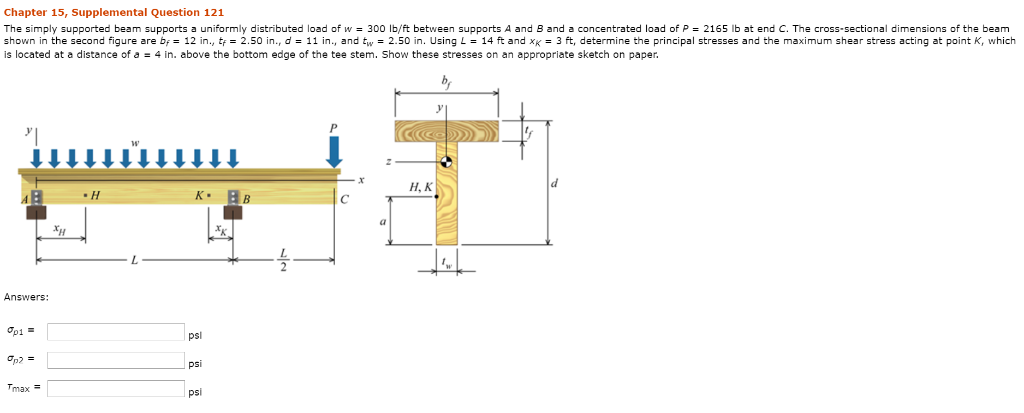Chapter 15, Supplemental Question 121 The simply supported beam supports a uniformly distributed load of w-300 lb/ft between supports A and B and a concentrated load of P = 2165 lb at end C. The cross-sectional dimensions of the beam shown in the second figure are b 12 in., t2.50 in.,d-11 in., and tw-2.50 in. Using L-14 ft and xK3 ft, determine the principal stresses and the maximum shear stress acting at point K, which is located at a distance...

• ### A Simply supported wood beam AB with span length Labm Carries trapezoidal distributed load of eatersita...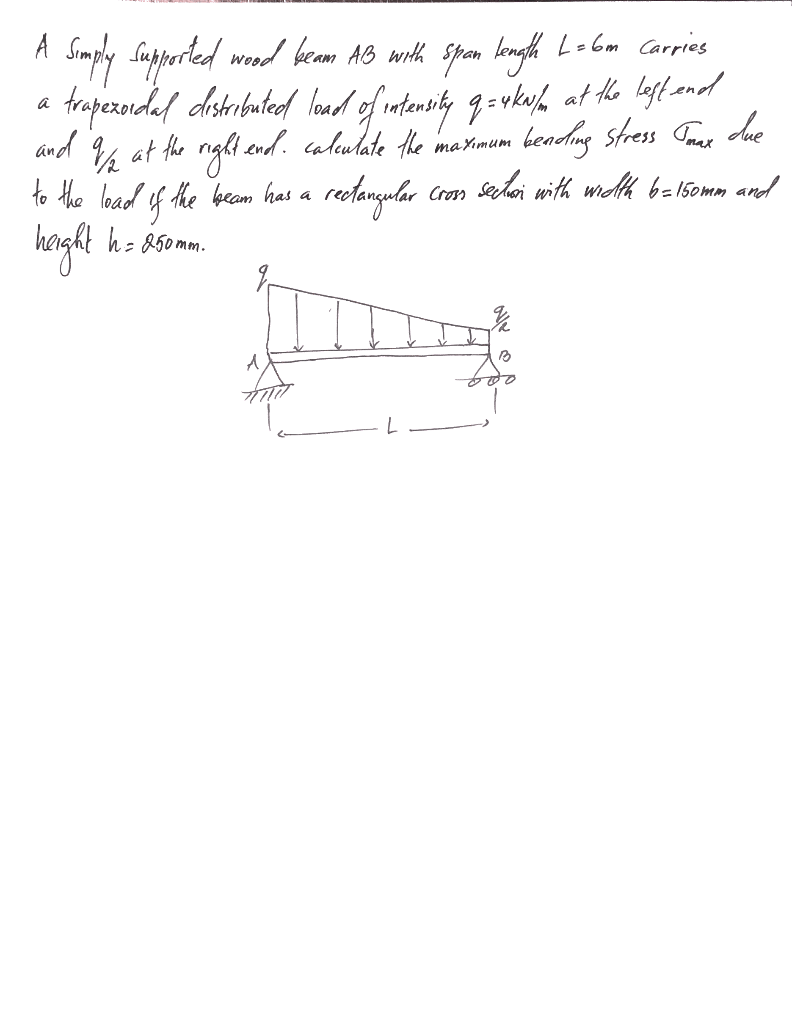A Simply supported wood beam AB with span length Labm Carries trapezoidal distributed load of eatersita q=vikula at the best and and at the right end. calculate the maximum bending stress Tomax clue to the load of the beam has a rectangular sechan with width 6=150mm and 9/2 Cross height ho 850mm. a

• ### A simply supported beam has a cross section of 2 in. wide by 6 in. tall....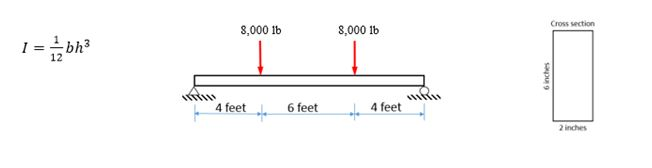A simply supported beam has a cross section of 2 in. wide by 6 in. tall. It is loaded by two vertical 8,000 lb forces acting at 4 ft from the ends of the beam. a.)    Determine the maximum bending stress, psi, in the beam b.)    Determine the maximum transverse shear stress, in psi, in the beam Cross section 8,000 lb 8,000 16 bh3 12 II 4 feet 6 feet 4 feet 2 inches

• ### A simply supported wood beam of rectangular cross section and span length 2 m carries a...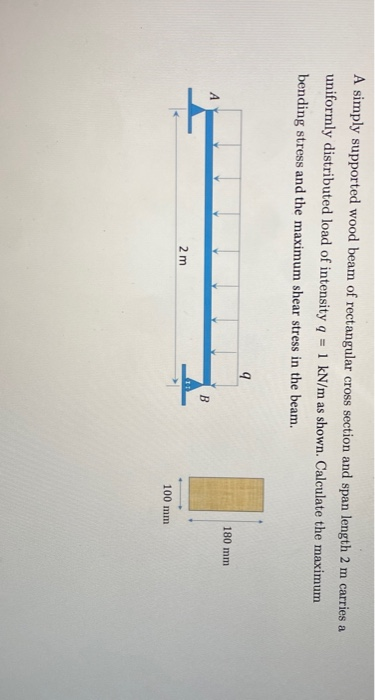A simply supported wood beam of rectangular cross section and span length 2 m carries a uniformly distributed load of intensity 9 = 1 kN/m as shown. Calculate the maximum bending stress and the maximum shear stress in the beam. 9 180 mm А B 2 m 100 mm

Free Homework App